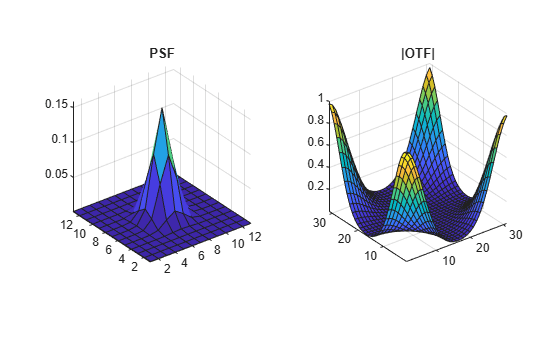# psf2otf

Convert point-spread function to optical transfer function

## Syntax

``OTF = psf2otf(PSF)``
``OTF = psf2otf(PSF,sz)``

## Description

example

````OTF = psf2otf(PSF)` computes the fast Fourier transform (FFT) of the point-spread function (`PSF`) array and creates the optical transfer function array, `OTF`, that is not influenced by the `PSF` off-centering.```
````OTF = psf2otf(PSF,sz)` specifies the size, `sz`, of the optical transfer function.```

## Examples

collapse all

`PSF = fspecial('gaussian',13,1);`

Convert the PSF to an Optical Transfer Function (OTF).

`OTF = psf2otf(PSF,[31 31]);`

Plot the PSF and the OTF.

```subplot(1,2,1); surf(PSF); title('PSF'); axis square; axis tight subplot(1,2,2); surf(abs(OTF)); title('Corresponding |OTF|'); axis square; axis tight```## Input Arguments

collapse all

Point-spread function, specified as a numeric array of any dimension.

Example: `PSF = fspecial('gaussian',13,1);`

Data Types: `single` | `double` | `int8` | `int16` | `int32` | `int64` | `uint8` | `uint16` | `uint32` | `uint64`
Complex Number Support: Yes

Size of the output optical transfer function `OTF`, specified as a vector of positive integers. The size of `OTF` must not exceed the size of `PSF` in any dimension. By default, `OTF` is the same size as `PSF`.

Data Types: `double`

## Output Arguments

collapse all

Optical transfer function, returned as a numeric array of size `sz`.

Data Types: `double`
Complex Number Support: Yes

## Tips

• To ensure that `OTF` is not altered because of `PSF` off-centering, `psf2otf` postpads `PSF` (down or to the right) with `0`s to match dimensions specified in `sz`. Then `psf2otf` circularly shifts the values of `PSF` up (or to the left) until the central pixel reaches (1,1) position.

• This function is used in image convolution and deconvolution when the operations involve the FFT.

## Version History

Introduced before R2006a